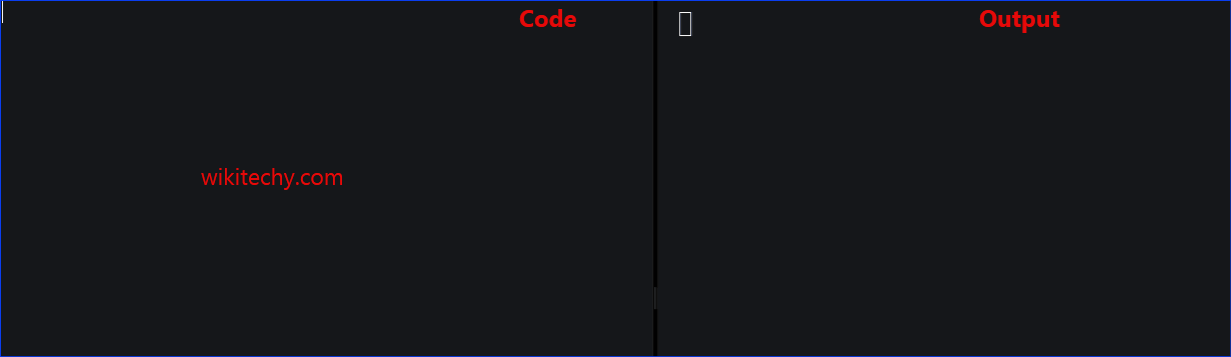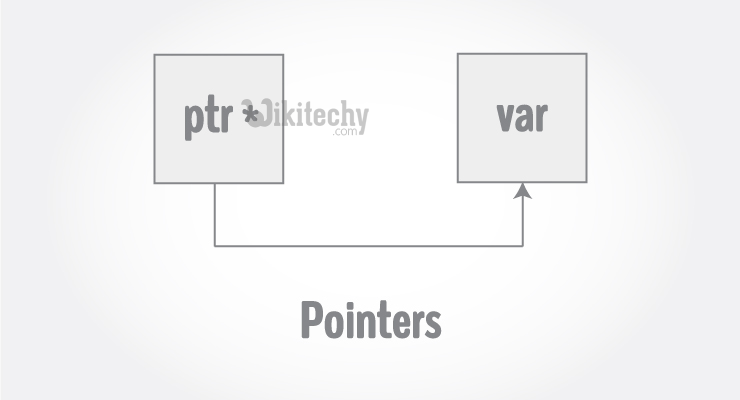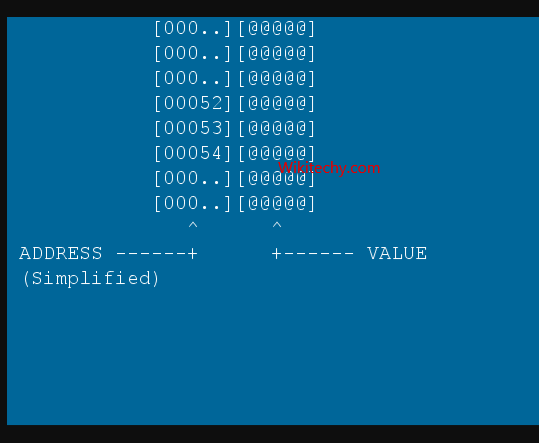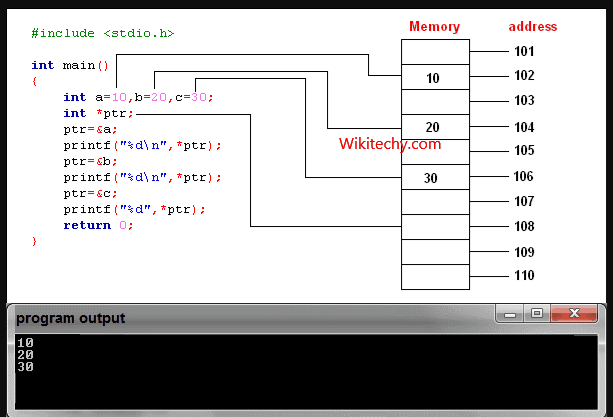# C++ Pointers - Learn C++ - C++ Tutorial - C++ programmingLearn c++ - c++ tutorial - c++-pointers - c++ examples - c++ programs• Pointers are powerful features of C++ that differentiates it from other programming languages like Java and Python.
• Pointers are used in C++ program to access the memory and manipulate the address.
###### Learn C++ , C++ Tutorial , C++ programming - C++ Language -Cplusplus

• To understand pointers, you should first know how data is stored on the computer.
• Each variable you create in your program is assigned a location in the computer's memory. The value the variable stores is actually stored in the location assigned.
• To know where the data is stored, C++ has an & operator. The & (reference) operator gives you the address occupied by a variable.
• If var is a variable then, &var gives the address of that variablelearn c++ tutorials - pointers in c++ Example

## Output

Note: You may not get the same result on your system.

• The 0x in the beginning represents the address is in hexadecimal form.
• Notice that first address differs from second by 4-bytes and second address differs from third by 4-bytes.
• This is because the size of integer (variable of type int) is 4 bytes in 64-bit system

## Pointers Variables

• C++ gives you the power to manipulate the data in the computer's memory directly. You can assign and de-assign any space in the memory as you wish. This is done using Pointer variables.
• Pointers variables are variables that points to a specific address in the memory pointed by another variable.
•learn c++ tutorials - pointers in c++ Example

## How to declare a pointer?

• The statement above defines a pointer variable p. It holds the memory address
• The asterisk is a dereference operator which means pointer to.
• Here, pointer p is a pointer to int, i.e., it is pointing to an integer value in the memory address.

## Reference operator (&) and Deference operator (*)

• Reference operator (&) as discussed above gives the address of a variable.
• To get the value stored in the memory address, we use the dereference operator (*).
• For example: If a number variable is stored in the memory address 0x123, and it contains a value 5.
• The reference (&) operator gives the value 0x123, while the dereference (*) operator gives the value 5.

## Example 2: C++ Pointers

• C++ Program to demonstrate the working of pointer.

## Output## Explanation of program

• When c = 5; the value 5 is stored in the address of variable c - 0x7fff5fbff8c.
• When pc = &c; the pointer pc holds the address of c - 0x7fff5fbff8c, and the expression (dereference operator) *pc outputs the value stored in that address, 5.
• When c = 11; since the address pointer pc holds is the same as c - 0x7fff5fbff8c, change in the value of c is also reflected when the expression *pc is executed, which now outputs 11.
• When *pc = 2; it changes the content of the address stored by pc - 0x7fff5fbff8c. This is changed from 11 to 2. So, when we print the value of c, the value is 2 as well.

## Common mistakes when working with pointers

• Suppose, you want pointer pc to point to the address of c. Then,
• In both cases, pointer pc is not pointing to the address of c.